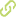# On Process Equivalence = Equation Solving in CCS•
• Overview
•
• Identity
•
•
• View All
•

### Abstract

• Unique Fixpoint Induction (UFI) is the chief inference rule to prove the equivalence of recursive processes in the Calculus of Communicating Systems (CCS) (Milner 1989). It plays a major role in the equational approach to verification. Equational verification is of special interest as it offers theoretical advantages in the analysis of systems that communicate values, have infinite state space or show parameterised behaviour. We call these kinds of systems VIPSs. VIPSs is the acronym of Value-passing, Infinite-State and Parameterised Systems. Automating the application of UFI in the context of VIPSs has been neglected. This is both because many VIPSs are given in terms of recursive function symbols, making it necessary to carefully apply induction rules other than UFI, and because proving that one VIPS process constitutes a fixpoint of another involves computing a process substitution, mapping states of one process to states of the other, that often is not obvious. Hence, VIPS verification is usually turned into equation solving (Lin 1995a). Existing tools for this proof task, such as VPAM (Lin 1993), are highly interactive. We introduce a method that automates the use of UFI. The method uses middle-out reasoning (Bundy et al. 1990a) and, so, is able to apply the rule even without elaborating the details of the application. The method introduces meta-variables to represent those bits of the processes' state space that, at application time, were not known, hence, changing from equation verification to equation solving. Adding this method to the equation plan developed by Monroy et al. (Autom Softw Eng 7(3):263-304, 2000a), we have implemented an automatic verification planner. This planner increases the number of verification problems that can be dealt with fully automatically, thus improving upon the current degree of automation in the field. © 2009 Springer Science+Business Media B.V.

• June 1, 2009